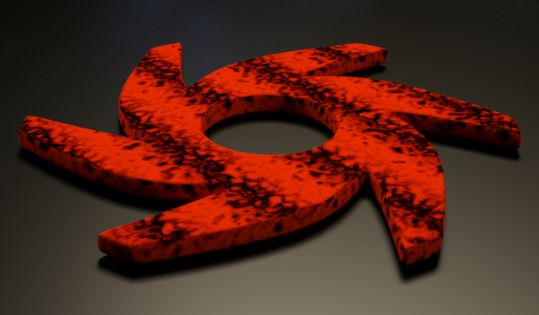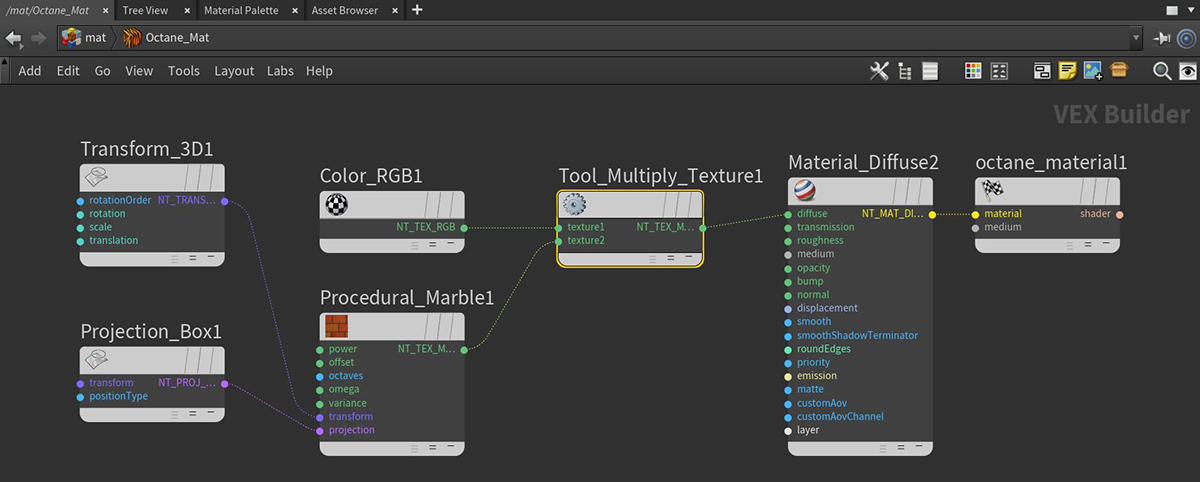# Multiply Texture

The Multiply texture multiplies the values of textures or colors together on two incoming Texture inputs. Black areas of the textures produces black, and white areas of the textures leave the mix unchanged.

In Figure 1, a red RGB color node is multiplied with a Marble node.Figure 1: A red RGB node multiplied with a Marble nodeFigure 2: Multiply node network